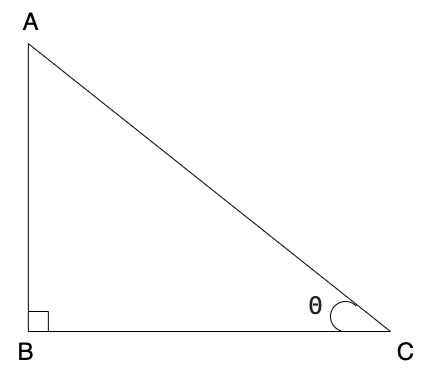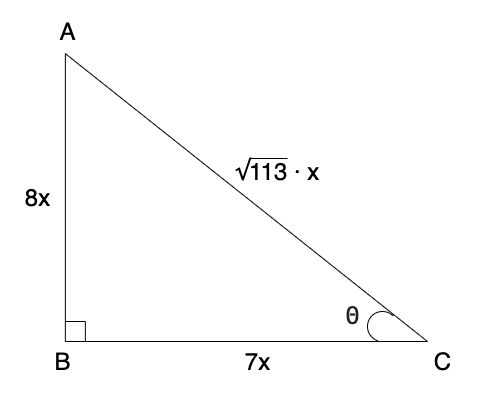Dark mode: OFF

# cot θ = 7/8 | Find all other trigonometric ratios | Trigonometry Numerical

We have already studied the basics of Trigonometry and the formulas of Trigonometry in the previous notes about Trigonometry. If you haven't read the notes, read it now

## Question: If $\ cot \theta = {7 \over 8}$; then find all other trigonometric ratios.

Given that, $cot \theta = {7 \over 8}$

As shown in the figure below, let's assume that we have a right triangle $\triangle ABC$, where $m \angle B = 90 \degree$Now from the formula of $cot \theta$, that we learned in this notes, we know that:

$cot \ \theta = {\text{Adjacent side to } \theta \over \text{Opposite side to } \theta}$

Putting values in this equation and solving further,

\begin{aligned} \therefore cot \theta &= {BC \over AB} \\ \therefore {7 \over 8} &= {BC \over AB} \\ \end{aligned}

From the equation above, let
$AB = 8 \sdot x$
$BC = 7 \sdot x$

Putting the values of $AB$ and $BC$ in the figure with $\triangle ABC$Now,
By Pythagoras theorem

\begin{aligned} AC^2 &= AB^2 + BC^2 \\ &= (8x)^2 + (7x)^2 \\ &= 64x^2 + 49x^2 \\ \therefore AC^2 &= 113x^2 \\ \therefore AC &= \sqrt {113x^2} \\ &= \sqrt {113} \sdot x \end{aligned}

Now, that we have the values of $AB$, $BC$ and $AC$ we can find the values of $sin \ \theta$, $cos \ \theta$, $tan \ \theta$, $cosec \ \theta$ and $sec \ \theta$ by applying the formulas that we learnt in the basics of trigonometry#### For $sin \ \theta$,

\begin{aligned} sin \ \theta &= {\text {Opposite side to } \theta \over Hypotenuse} \\ \\ &= {AB \over AC} \\ \\ &= {8x \over \sqrt {113} \sdot x} \\ \\ &= {8 \cancel{x} \over \sqrt {113} \sdot \cancel{x}} \\ \\ & \boxed {\therefore sin \ \theta = {8 \over \sqrt {113}}} \\ \end{aligned}

#### For $cos \ \theta$,

\begin{aligned} cos \ \theta &= {\text {Adjacent side to } \theta \over Hypotenuse} \\ \\ &= {BC \over AC} \\ \\ &= {7x \over \sqrt {113} \sdot x} \\ \\ &= {7 \cancel{x} \over \sqrt {113} \sdot \cancel{x}} \\ \\ & \boxed {\therefore cos \ \theta = {7 \over \sqrt {113}}} \\ \end{aligned}

#### For $tan \ \theta$,

\begin{aligned} tan \ \theta &= {sin \ \theta \over cos \ \theta} \\ \\ &= {1 \over cot \ \theta} \\ \\ &= {1 \over {7 \over 8}} \\ \\ &= {8 \over 7} \\ \\ & \boxed {\therefore \tan \ \theta = {8 \over 7}} \end{aligned}

#### For $sec \ \theta$,

\begin{aligned} sec \ \theta &= {1 \over cos \ \theta} \\ \\ &= {1 \over {7 \over \sqrt {113}}} \\ \\ &= {\sqrt {113} \over 7} \\ \\ &\boxed {\therefore sec \ \theta = {\sqrt {113} \over 7}} \end{aligned}

#### For $cosec \ \theta$,

\begin{aligned} cosec \ \theta &= {1 \over sin \ \theta} \\ \\ &= {1 \over {8 \over \sqrt {113}}} \\ \\ &= {\sqrt {113} \over 8} \\ \\ &\boxed {\therefore cosec \ \theta = {\sqrt {113} \over 8}} \end{aligned}

### Help us build Education Lessons

If our notes and videos are helpful to you, kindly support us by making a donation from our support page so we can continue making more content for students like you.

Go to support page